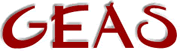[ < ] [ > ] [ << ] [ Up ] [ >> ] [Top] [Contents] [Index] [ ? ]

#### 1.2.7.3 Boolean operators

Boolean (binary) operators are applicable only to integers with the exception of the `&` operator which also works on arrays. Internally an integer is 32 bits long. However, in the following examples I will only show the ten last bits as the others are 0 and can be ignored with the one exception of the `~`-operator.

1. E1 & E2 E1 and E2.

 ```1011101001 (= 745) 1000100010 & (= 546) ------------ 1000100000 (= 544) => 745 & 546 = 544 ```

Used on two arrays, this function will return a new array that holds all elements that are members of both of the argument arrays.

2. E1 | E2 E1 or E2.

 ```1011101001 (= 745) 1000100010 | (= 546) ------------ 1011101011 (= 747) => 745 | 546 = 747 ```

3. E1 ^ E2 E1 xor (exclusive or) E2.

 ```1011101001 (= 745) 1000100010 ^ (= 546) ------------ 0011001011 (= 203) => 745 ^ 546 = 203 ```

4. ~E 1-complement of E (invert E).

 ```00000000000000000000001011101001 ~ (= 745) ---------------------------------- 11111111111111111111110100010110 (= -746) => ~745 = -746 ```

NB! The above example might be hard to understand unless you really know your binary arithmetic. However, trust me when I say that this is not a typo, it's the way it should look. Read a book on boolean algebra (the section on two-complement binary arithmetic) and all will be clear.

5. E1 << E2 E1 is shifted left E2 steps.

 ```5 << 4 => 101(b) << 4 = 1010000(b) = 80 ```

6. E1 >> E2 E1 is shifted right E2 steps.

 ```1054 >> 5 => 10000011110(b) >> 5 = 100000(b) = 32 ```

This document was generated by Ronny Wikh on July, 8 2003 using texi2html